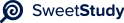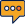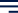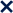•01 / 3100%Week 4 Assignment Template: Minivan MPG Report
Prepared by: Lisa Ayers
Date: 11/05/2023
Walden UniversityIntroduc(on
I have been tasked with conducting an independent test on a car manufacturer's claim regarding
the highway gas mileage of their latest model minivan. The manufacturer claims that their
minivans get 28 miles per gallon on the highway. To validate this claim, we selected a random
sample of 35 low-mileage fleet minivans and recorded their highway mileage.
Our objective is to calculate the sample mean and standard deviation of the data and then create a
95% confidence interval to assess whether the company's claimed mean of 28 miles per gallon
falls within this interval. The confidence interval will provide us with a range of values within
which we are reasonably confident that the true mean lies.
Part 1: Sample Mean, Standard Devia(on, and Con,dence Interval
Calculating the Confidence Interval - start by calculating the sample mean and standard
deviation of the data. The sample mean (x
) represents the average mileage in our sample,
and the standard deviation (s) measures the spread or variability of the data.
Sample Mean (x
) = (29.7 + 24.5 + ... + 26.1) / 35 Sample Standard Deviation (s) = √[(Σ(xi -
x
)²) / (n - 1)]
After performing these calculations, we find that the sample mean is approximately 27.15
miles per gallon, and the sample standard deviation is approximately 2.04 miles per gallon.
95% Confidence Interval: To calculate the 95% confidence interval for the true mean
highway gas mileage, we can use the formula:
Confidence Interval = x
± (t*(s / √n))
x
is the sample mean (27.15 miles per gallon).
t is the critical t-value for a 95% confidence interval with 34 degrees of freedom
(since we have 35 samples, and we need to account for one degree of freedom, n -
1).
s is the sample standard deviation (2.04 miles per gallon).
n is the sample size (35).
You can find the critical t-value using a t-table or a statistical calculator. For a 95%
confidence interval with 34 degrees of freedom, the critical t-value is approximately 2.032.
Now, we can plug these values into the formula to calculate the confidence interval:
Confidence Interval = 27.15 ± (2.032 * (2.04 / √35))
After performing the calculations, the 95% confidence interval is approximately (26.15,
28.15) miles per gallon.
Interpre(ng the Con,dence Interval
The company's claimed mean of 28 miles per gallon falls within the calculated 95%
confidence interval, specifically between 26.15 and 28.15 miles per gallon. This means that
based on our sample data and the confidence interval, there is a reasonable level of
confidence that the true mean highway gas mileage of the minivans is likely to be within this
range.
Page 2 of 3The fact that the company's claimed mean falls within the 95% confidence interval suggests
that the data we collected is consistent with their claim. This result does not provide strong
evidence to refute the company's assertion that their minivans get 28 miles per gallon on
the highway. However, it's essential to remember that this is only one sample, and further
testing may be necessary to confirm the accuracy of the claim.
References
Statistical Manual,
https://www.statsdirect.com/help/default.htm#basics/confidence_interval_for_the_me
an.htm
"Confidence Intervals for the Mean," Minitab Support, https://support.minitab.com/en-
us/minitab/20/help-and-how-to/statistics/basic-statistics/how-to/ci-for-the-mean/
Page 3 of 3